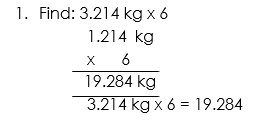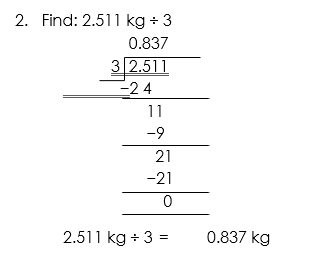# WEIGHT

##### HELLO, WELCOME BACK TO CLASS

When you mention how heavy or light an object is, you are referring to its weight. In the.

We know the main standard unit of mass or weight is kilogram which we write in short as ‘kg’. 1000th part of this kilogram is gram which is written in short as ‘g’.

Thus 1000 gram = 1 kilogram and 1 kilogram = 1000 gram

i.e. 1000 g = 1 kg and 1 kg = 1000 g.

This gram (g) is a very small unit of mass.

We use other units of mass or weight to conveniently measure the mass or weight of materials.

 1/2 of 1 kg   = 500 grams 1/4 of 1 kg   = 250 grams 1/5 of 1 kg   = 200 grams 1/10 of 1 kg = 100 grams 1/20 of 1 kg =   50 grams or           2 x 500 grams   = 1 kg or           4 x 250 grams   = 1 kg or           5 x 200 grams   = 1 kg or           10 x 100 grams = 1 kg or           20 x 50 grams  = 1 kg

One kilogram is the measure of mass of an object having one kilogram of weight. Its abbreviation is kg. Grams abbreviation is g.

1 kg = 1000 g

or, 1000 g = 1 kg

Gram is a part of one kilogram, if it is divided in 1000 equal parts.

½ kg = 500 g

or, 500 g = ½ kg

500 grams weight is half of one kilogram.

250 g = ¼ kg

250 grams weight is one-fourth of one kilogram.

Say we have to buy some wheat from a shop. The shopkeeper weighs 5 kg once and then 3 kg. Find how much wheat we have bought.

First the shopkeeper weighs 5 kg of wheat

Then he weighs                        3 kg of wheat

Total amount of wheat   =       5 kg + 3 kg = 8 kg

Thus, we have to add or subtract masses of weights of objects.

1. Mary purchased 2 kg apples, 3 kg pears and 5 kg mangoes. Find the total weight of fruits purchased.

Solution:

Apples                   = 2 kg

Pears                    = 3 kg

Mangoes                = 5 kg

Therefore, total weight of fruits purchased = 2 kg + 3 kg + 5 kg = 10 kg fruits

1. (i) Subtract 453 grams from 765 grams

(ii) Convert 5 kg 300 g into g.

Solution:

(i)               765 grams

453 grams

312 grams

(ii) 5 kg 300 g = 5 × 1000 g + 300 g

= 5000 g + 300 g

= 5300 g

Problems on Weight involving Kilogram (kg) and Grams (g)

Definition: Weight is the amount somebody or something weighs- the heaviness of a particular object or person, especially as measured by a particular system of weight.

The units used for weight are kilograms (kg) and grams (g).

1000 grams (g) = 1 kilogram (kg)

Examples:WORD PROBLEMS ON WEIGHT

Examples:

1. How many 50 g packets of tea can be made from
2. 500 g   1 ½ kg                  c. 84 kg                     d.  9 kg                      e.  2 ¼ kg

Solution:

1. 500 g ÷ 50 g = 50 ÷5 = 10 packets
2. 1 ½ : Convert to grams

1 kg = 1000 g                 ½ kg = 1000 ÷ 2 = 500 g

1000 g + 500 g = 1500 g

1500 g ÷ 50 g = 150 ÷ 5 = 30 packets

1. 84 kg to g = 84 x 1000 = 84 000 g

84 000 ÷ 50 = 8 400 ÷ 5 = 1,680 packets

1. 9 kg to g = 9 x 1000 = 9 000 g

9 000 ÷ 50 = 900 ÷ 5 = 180 packets

1. 2 ¼ kg to g =2 000 + (¼ x 1 000) = 2 000 + 250 = 2250 g

2 250 ÷ 50 = 225 ÷ 5 = 45 packets

1. Simbi weighs 36 kg. Ada weighs 49 kg. Emeka weighs 32 kg and Ali weighs 35 kg. How much do the girls Simbi and Ada weigh more than the boys.

Solution:

Simbi weighs                                               36 kg

Simbi and Ada weigh                              85 kg

Emeka weighs                                           32 kg

Ali weighs                                               +   35 kg

Emeka and Ali weigh                               67 kg

Difference between the girls’ weight and the boys’ weight = 85 kg −67 kg = 17 kg.

Quiz

1. Ron purchased 60 kg sugar from one shop and 45 kg sugar from another shop. How much sugar did he purchase?
2. Sam had 75 kg of rice. He sold 25 kg of it. How much rice is left with him?
3. Aaron weighs 40 kg and Barun weighs 45 kg. What is their total weight?
4. Mike, David and Ken weight 30 kg, 25 kg, and 20 kg respectively. Find their total weight.
5. Jack produced 300 kg of rice in one field and 250 kg of rice in another field. How ore rice did he produces in the first field?
6. Robert weighs 32 kg 350 g and Joseph 30 kg 200 gm. What is their total weight? Find who is heavier and by how much.

(i) 12 kg + 8 kg = ____ kg

(ii) 22 kg + 25 kg = ____ kg

(iii) 75 kg + 22 kg = ____ kg

(iv) 530 g + 437 g = ____ g

(v) 378 g + 211 g = ____ g

(vi) 3 kg 350 g + 5 kg 230 g = ____ kg ____ g

1. Subtract:

(i) 15 kg – 7 kg = ____ kg

(ii) 27 kg – 17 kg = ____ kg

(iii) 48 kg – 25 kg = ____ kg

(iv) 825 g – 203 g = ____ g

(v) 325 g – 210 g = ____ g

(vi) 5 kg 480 g – 2 kg 378 g = ____ kg ____ g

How Can We Make ClassNotesNG Better? - CLICK to Tell Us Now!